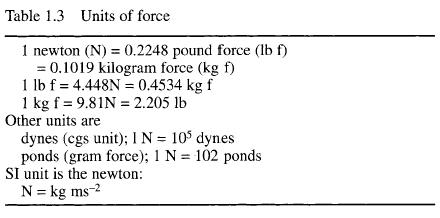﻿ Definition of terms, Mass and force – Hydraulic Schematic Troubleshooting

## Definition of terms, Mass and force

Pneumatic and hydraulic systems generally rely on pressure in a fluid. Before we can discuss definitions of pressure, though, we must first be clear what is meant by everyday terms such as weight, mass and force.

We all are used to the idea of weight, which is a force arising from gravitational attraction between the mass of an object and the earth. The author weighs 75 kg on the bathroom scales; this is equivalent to saying there is 75 kg force between his feet and the ground.

Weight therefore depends on the force of gravity. On the moon, where gravity is about one sixth that on earth, the author’s weight would be about 12.5 kg; in free fall the weight would be zero. In all cases, though, the author’s mass is constant.

The British Imperial fps system and the early metric systems link mass and weight (force) by defining the unit of force to be the gravitational attraction of unit mass at the surface of the earth. We thus have a mass defined in pounds and force defined in pounds force (lbs f) in the fps system, and mass in kilogrammes and force in kg f in the mks system.

Strictly speaking, therefore, bathroom scales which read 75 kg are measuring 75 kg f, not the author’s mass. On the moon they would read 12.5 kg f, and in free fall they would read zero.

If a force is applied to a mass, acceleration (or deceleration) will result as given by the well known formula:

F = ma.                         (1.1)

Care must be taken with units when a force F is defined in lbs f or kg f and mass is defined in lbs or kg, because resulting accelerations are in units of g; acceleration due to gravity. A force of 25 kg f applied to the author’s mass of 75 kg produces an acceleration of 0.333 g.

The SI unit of force, the newton (N), is defined not from earth’s gravity, but directly from expression 1.1. A newton is defined as the force which produces an acceleration of 1 m s -2 when applied to a mass of 1 kg.

One kgf produces an acceleration of 1 g (9.81 ms -z) when applied to a mass of 1 kg. One newton produces an acceleration of 1 ms -2 when applied to mass of 1 kg. It therefore follows that:

1 kgf=9.81 N

but as most instruments on industrial systems are at best 2% accurate it is reasonable (and much simpler) to use:

1 kgf=10N

for practical applications.

Table 1.3 gives conversions between various units of force.